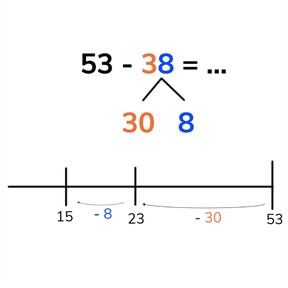Subtraction to 100 by splitting the subtrahend with subtrahends >10

# Subtraction to 100 by splitting the subtrahend with subtrahends >10

No account needed.8,000 schools use Gynzy92,000 teachers use Gynzy1,600,000 students use Gynzy

## General

Students learn to subtract by splitting the subtrahend into smaller number(s) and taking it away from the first number in steps.

2.NBT.B.5

## Relevance

Discuss with students that splitting the subtrahend is useful when you can't take away the numbers in one time in your head. An example would be if you want to know how many miles Mike still needs to ride to make 76 if they've already biked 28 miles.

## Introduction

Divide the class into groups. Give a number to the class, in the group, each member must say the next number back out loud. Start the timer. When the timer runs out, ask students how far they got. Next show students a number line which shows different jumps back on the number line. Ask students which numbers belong at the grey squares. Erase the squares to show the answers.

## Development

Explain to students that splitting the subtrahend means that you divide the subtrahend into smaller parts that are easier to take away from the first number (the minuend). Then remind students that tens are a group of 10 and end on a zero and that ones are the numbers 1 to 9. Explain that when using a number line to subtract by splitting the subtrahend, you place the first number at the end of the number line. Then you jump back by the tens and then ones that you split your subtrahend into. You can also decide to first jump back to the previous tens number, and then jump back by tens and ones. Where did you end on the number line? Have students determine the number and explain that this is your difference. Practice two more subtraction problems on the number line, one where the jumps are shown on the number line, and the last in which students are asked to determine which jumps to take. Next tell students that you can subtract by splitting the subtrahend even when you don't use a number line. You do so by splitting the subtrahend into tens and ones and then taking them away from the first number (the minuend). You first subtract the tens, and then subtract the ones from that difference. Remind students that they can always split their ones again to subtract to a tens number to make subtraction easier. Ask students which steps they take to subtract two given numbers and practice splitting the subtrahend. Ask students to explain their strategy. Erase the grey squares to check their answers.

Check that students are able to subtract numbers to 100 by splitting the subtrahend with subtrahends greater than 10 by asking the following questions:
- How do you subtract by splitting the subtrahend?
- Why is it useful to split the subtrahend?

## Guided practice

Students are first given problems with support of a number line, then are asked to solve without a number line. Students also are given some subtraction problems with the subtrahend already split for them, but later must split the subtrahend themselves.

## Closing

Discuss with students that it is important to be able to subtract numbers to 100 by splitting the subtrahend with subtrahends greater than ten, so you can easily subtract larger numbers. Ask students to form pairs and solve the given subtraction problem on their own number lines. Have the pairs compare the jumps they took/compare how they split their subtrahends. Emphasize that students are free to make the jumps that help make the problem easier for them, and that that often is by tens and ones as well as jumping to the previous tens number to form a round number. Then have the students form groups of 4/5. Give each student a number card. Tell the students that the student with the largest number is the minuend (the first number of the subtraction problem) and that every other person in the group must form a subtraction problem with that largest number and they must solve each subtraction problem as a group.

## Teaching tips

Students who have difficulty subtracting by splitting the subtrahend can be supported by the use of a number line. Have them draw out all of the jumps and steps beween the two numbers. Encourage them to split the subtrahend in a way that makes it easy for them to subtract (so back to the previous tens number, then by tens, and the rest of the ones). Students can also use manipulatives to represent the minuend, so they can tangibly split the minuend.

## Instruction materials

Number cards to 100.

### The online teaching platform for interactive whiteboards and displays in schools

• Save time building lessons

• Manage the classroom more efficiently

• Increase student engagement